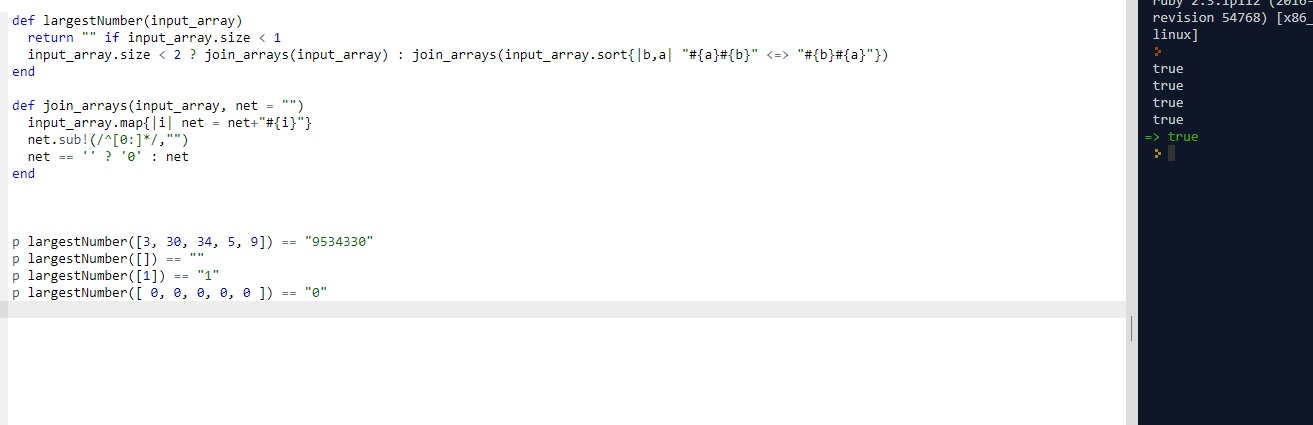# CLEAN CODE: LARGEST NUMBER

By: Saurav

2017-12-19 10:13:00 UTC

Given a list of non negative integers, arrange them such that they form the largest number.

For example:

Given [3, 30, 34, 5, 9], the largest formed number is 9534330.

Note: The result may be very large, so you need to return a string instead of an integer.

InterviewBit

This problem is challenging in the sense you need to know how sorting works at the basic level.

One simple way can be to iterate through each number in an array and place it at every available place, store the number generated in an array and then get the maximum of all. Clearly this method is O(n^2) and we want better.

Recently I was asked in an interview to sort an array of points (with x,y coordinates) with respect to the distance from the origin.
It was another variation of sorting with respect to object's property so these types of problems are very fresh in my mind.

Approach:
What if we change our normal sorting function where instead of comparing the actual value of two numbers, we compare the numbers generated by two arrangements:
ab and ba for two numbers a and b

Lets start by writing some tests and then the first draft:

```p largestNumber([3, 30, 34, 5, 9]) == "9534330"
p largestNumber([]) == ""
p largestNumber() == "1"
p largestNumber([ 0, 0, 0, 0, 0 ]) == "0"
```

```def largestNumber(input_array)
input_array.size < 2 ? join_arrays(input_array) : join_arrays(input_array.sort{|b,a| "#{a}#{b}" <=> "#{b}#{a}"}).to_i.to_s
end

def join_arrays(input_array, net = "")
input_array.map{|i| net = net+"#{i}"}
net
end
```

Which works fine and is clean.

But as per interview bit, the integer generated would be very large and may overflow. We may need to change our function to handle the case of many zeroes specifically.

```def largestNumber(input_array)
return "" if input_array.size < 1
input_array.size < 2 ? join_arrays(input_array) : join_arrays(input_array.sort{|b,a| "#{a}#{b}" <=> "#{b}#{a}"})
end

def join_arrays(input_array, net = "")
input_array.map{|i| net = net+"#{i}"}
net.sub!(/^[0:]*/,"")
net == '' ? '0' : net
end
```As we see, all our tests pass and our code looks clean as well.

### Let me know what you think! Twitter: sprakash24octLinkedin

Owned & Maintained by Saurav Prakash

If you like what you see, you can help me cover server costs or buy me a cup of coffee though donation :)